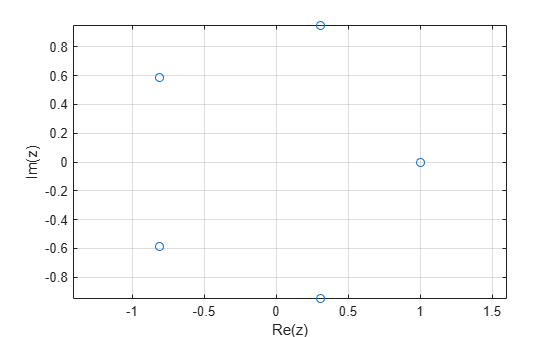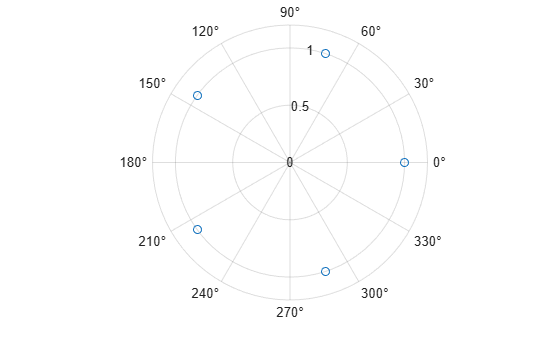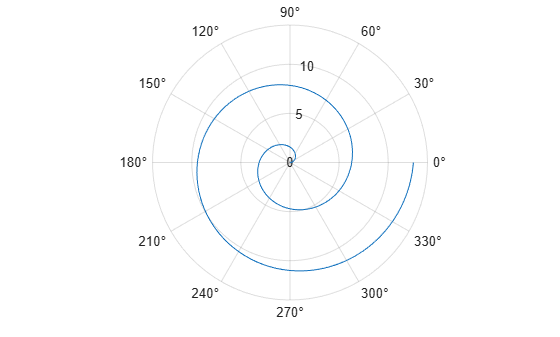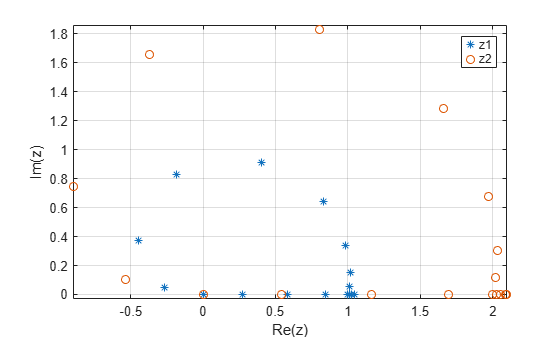# Plot Complex Numbers

This example shows how to plot complex numbers in MATLAB®. A complex number $z$ is a number that can be written in the form

$\mathit{z}=\mathit{x}+\mathit{y}\text{\hspace{0.17em}}\mathrm{i}$,

where $x$ and $y$ are real numbers, and $i$ is the imaginary unit, which is defined as ${i}^{2}=-1$. The number $x$ is the real part of the complex number, which is denoted by $x=Re\left(z\right)$, and the number $y$ is the imaginary part of the complex number, which is denoted by $y=Im\left(z\right)$. You can plot a complex number as a pair of coordinates $\left(x,y\right)$ on the complex plane, also known as the Argand diagram. This diagram uses the Cartesian coordinates to represent the real part in the $x$-axis and the imaginary part in the $y$-axis.

You can also represent a complex number using the polar representation. The complex number is written in the form

$\mathit{z}=\mathit{r}\text{\hspace{0.17em}}{\mathit{e}}^{\mathit{i}\theta }=\mathit{r}\left(\mathrm{cos}\text{\hspace{0.17em}}\theta +\mathrm{i}\text{\hspace{0.17em}}\mathrm{sin}\text{\hspace{0.17em}}\theta \right)$,

where $r$ is the absolute value or magnitude of the complex number, and $\theta$ is the phase angle of the complex number. In this representation, you can plot a complex number as a point in the polar coordinates with radius $r$ (the distance from the origin) and polar angle $\theta$ (the counterclockwise angle between the positive real axis and the line connecting the point to the origin).

### Plot Array of Complex Numbers

Create a vector that contains the complex numbers `3 + 4i`, `-4 - 3i`, `1 - 2i`, and `-1 - 1i`.

`z = [3 + 4i; -4 - 3i; 1 - 2i; -1 - 1i]`
```z = 4×1 complex 3.0000 + 4.0000i -4.0000 - 3.0000i 1.0000 - 2.0000i -1.0000 - 1.0000i ```

Plot the imaginary part against the real part of the complex vector `z` by using `plot`. Use the `real` function and `imag` function to return the real and imaginary parts of the complex vector, respectively.

```plot(real(z),imag(z),"o") axis equal grid on xlabel("Re(z)") ylabel("Im(z)")```You can also use `plot(z,LineSpec)` instead of `plot(real(z),imag(z),LineSpec)` to plot an array of complex numbers. This function automatically plots the real part in the $x$-axis and the imaginary part in the $y$-axis.

### Plot Complex Roots of Unity in Cartesian Coordinates

The $n$th roots of unity are complex numbers that satisfy the polynomial equation

${\mathit{z}}^{\mathit{n}}=1$,

where $n$ is a positive integer.

The $n$th roots of unity are

$\mathrm{exp}\left(\frac{2\mathit{k}\pi \mathrm{i}}{\mathit{n}}\right)=\mathrm{cos}\frac{2\mathit{k}\pi }{\mathit{n}}+\mathrm{i}\text{\hspace{0.17em}}\mathrm{sin}\frac{2\mathit{k}\pi }{\mathit{n}}$, for $\mathit{k}=0,1,\dots ,\mathit{n}-1$.

To find the complex roots of unity, you can solve the polynomial equation by using `roots`. The `roots` function solves polynomial equations of the form ${\mathit{p}}_{1}{\mathit{x}}^{\mathit{n}}+\cdots +{\mathit{p}}_{\mathit{n}}\mathit{x}+{\mathit{p}}_{\mathit{n}+1}=0$. For example, find the fifth roots of unity of ${z}^{5}=1$, or ${z}^{5}-1=0$.

```p = [1 0 0 0 0 -1]; z = roots(p)```
```z = 5×1 complex -0.8090 + 0.5878i -0.8090 - 0.5878i 0.3090 + 0.9511i 0.3090 - 0.9511i 1.0000 + 0.0000i ```

Plot the complex roots of unity in the Cartesian coordinates.

```plot(z,"o") axis equal grid on xlabel("Re(z)") ylabel("Im(z)")```### Plot Complex Numbers in Polar Coordinates

Plot the fifth roots of unity in the polar coordinates by using `polarplot`. Use the `angle` function to return the phase angles of the complex roots, and use the `abs` function to return the absolute values or radii of the complex roots.

`polarplot(angle(z),abs(z),"o")`You can also use `polarplot(z,LineSpec)` instead of `polarplot(angle(z),abs(z),LineSpec)` to plot an array of complex numbers in the polar coordinates. This function automatically plots the radii and phase angles of the complex numbers.

### Plot Parametric Curve in Complex Plane

Define a parametric curve that has the form

`$z=f\left(t\right)=t\mathrm{exp}\left(it\right)$`

with the parameter $t$ in the interval $\left[0,4\pi \right]$.

Create a vector `t` of 200 equally spaced points within this interval to parameterize $t$. Define the points that lie on the complex curve as a complex vector `z`.

```t = linspace(0,4*pi,200); z = t.*exp(1i*t);```

Plot the complex curve in the Cartesian coordinates.

```plot(z,"-") axis equal grid on xlabel("Re(z)") ylabel("Im(z)")```Plot the complex curve in the polar coordinates.

`polarplot(z,"-")`### Plot Eigenvalues of Square Matrix

A real $n$-by-$n$ square matrix has $n$ eigenvalues (counting algebraic multiplicities) that either are real or occur in complex conjugate pairs.

For example, consider a 20-by-20 real matrix with random elements that are sampled from a standard normal distribution. Calculate the eigenvalues using `eig`.

```rng("default") z = eig(randn(20));```

Plot the imaginary part against the real part of all 20 eigenvalues. Notice that for each eigenvalue ${z}_{k}={x}_{k}+{y}_{k}\phantom{\rule{0.2222222222222222em}{0ex}}i$ that is not on the real axis, there is another complex conjugate pair of this eigenvalue ${z}_{k}^{*}={x}_{k}-{y}_{k}\phantom{\rule{0.2222222222222222em}{0ex}}i$.

```plot(z,"o") axis equal grid on xlabel("Re(z)") ylabel("Im(z)")```### Plot Multiple Complex Data Sets

Plot the imaginary part against the real part of two complex data sets. If you pass multiple complex input arguments to `plot`, such as `plot(z1,z2)`, then the `plot` function ignores the imaginary part and plots only the real part of the inputs. To plot the real part against the imaginary part for multiple complex inputs, you must explicitly pass the real part and the imaginary part to `plot`.

For example, create two complex vectors `z1` and `z2`.

```x = -2:0.25:2; z1 = x.^exp(-x.^2); z2 = 2*x.^exp(-x.^2);```

Find the real part and imaginary part of each vector by using the `real` and `imag` functions.

```re_z1 = real(z1); im_z1 = imag(z1); re_z2 = real(z2); im_z2 = imag(z2);```

Plot the complex data.

```plot(re_z1,im_z1,"*",re_z2,im_z2,"o") axis equal grid on legend("z1","z2") xlabel("Re(z)") ylabel("Im(z)")```